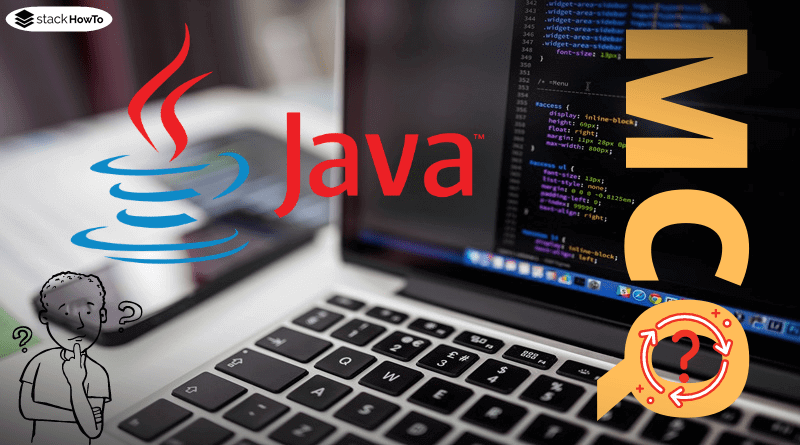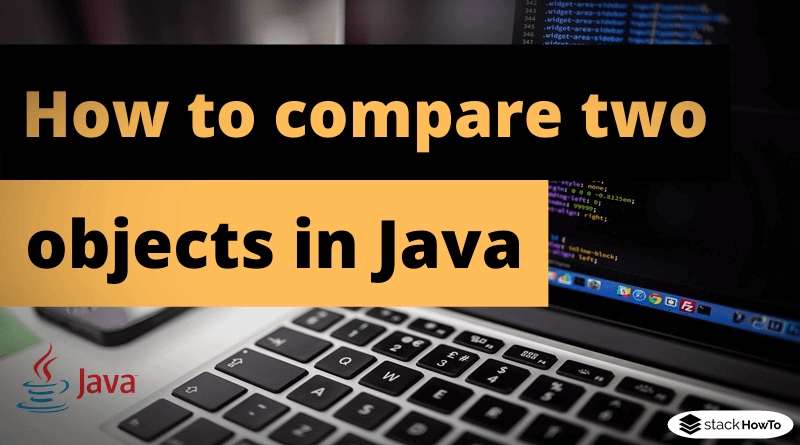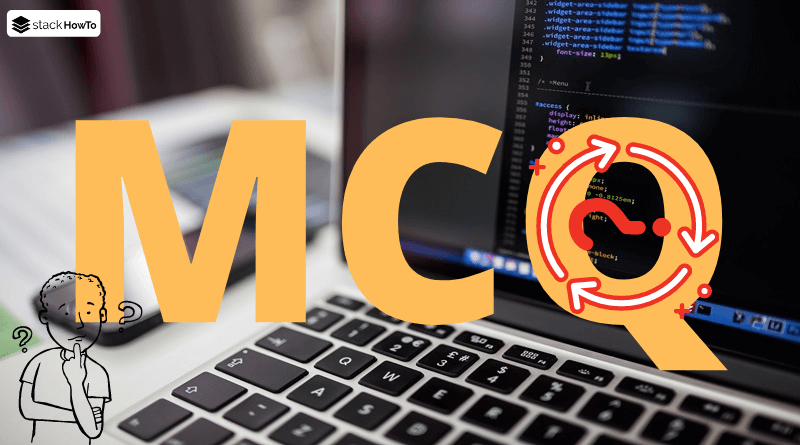java

# Difference between == and .equals()

In short: .equals() is used to compare objects, and the equal-to operator (==) is used to compare references and simple types such as int and boolean.

###### Comparison of primitive data types

Primitive or simple types such as int, double, long, float, etc. are not object types, but special forms of data types in Java. The primitive types have no properties or methods but only represent a value. Since there are no methods, there are of course no equals :).

```int x = 100;
int y = 100;
System.out.println(x == y);```

Output:

`true`

Similar to int, the other simple types are also compared with ==.

###### Comparison of references

If two variables are the same object, i.e. the reference to the same object, can be determined using the equal-to operator (==) too. We say that these objects have the same identity, so the equal-to operator (==) compares the identity.

```Person p1 = new Person("1", "Alex", "Foubier");
Person p2 = new Person("1", "Alex", "Foubier");
Person p3 = p1;

System.out.println(p1 == p2); // Display: false
System.out.println(p1 == p3); // Display: true```

Although p1 and p2 describe the same person, they are still 2 different objects (new always creates a new object!).Java MCQ – Multiple Choice Questions and Answers – OOPsThis collection of Java Multiple Choice Questions and Answers (MCQs): Quizzes & Practice Tests with Answer focuses on “Java OOPs”.   1. Which of the…Read More

###### Comparison using .equals()

Java is an object-oriented language. Each object can decide for itself whether it is the same as another or not, so each object has an equals(Object o) method. When you create a class, it inherits the functionality of equals from the superclass or Object. However, by default, this only compares for identity, which is often not desired. Therefore the equals method has to be overridden in many cases.

Important: if a class overwrites the method equals(), the method hashCode() must also be overwritten (but this is not a big problem, see below).

Here is an example of how equals and hashCode can be implemented:

```@Override
public boolean equals(Object o) {
if (this == o){
return true;
}
if (!(o instanceof Person)){
return false;
}

Person person = (Person) o;
return Objects.equals(this.id, person.id)
&& Objects.equals(this.firstName, person.firstName)
&& Objects.equals(this.lastName, person.lastName);
}```
• First, (this == o) is used to check if it is the same reference. If it is, the objects are of course the same, because it is the same object in memory
• Then (o instanceof person) is used to check whether the other object is of the same type or subclass. If not, the objects cannot be the same.
• Finally, every single field of the other object is compared with the own one. Only if all match, the objects are equal.

The implementation of the hashCode() function is quite simple thanks to the utility class Objects:

```@Override
public int hashCode() {
return Objects.hash(id, firstName, lastName);
}```

It is important to override the hashCode() method, otherwise some data structures like maps may not work or not work correctly with the class.How to compare two objects in JavaWhen are two objects “equal”? This question always causes confusion. The key to understanding it is to learn the difference between the relational operator ==…Read MoreMCQPractice competitive and technical Multiple Choice Questions and Answers (MCQs) with simple and logical explanations to prepare for tests and interviews.Read More